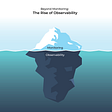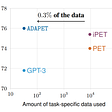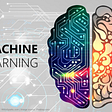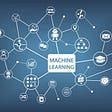# Machine Learning #2: Supervised Learning

In this post, we look at what is supervised learning and one of the most basic algorithms in supervised learning, Linear Regression.

# What is supervised learning?

In the last post, we saw how the essence of ML is to generate/modify the algorithm so that the output is maximally error-free.

Of the many categories of ML algorithms that exist, one of the most popular is the Supervised Learning Algorithm.

Here’s a definition of supervised learning from IBM’s blog

Supervised learning uses a training set to teach models to yield the desired output. This training dataset includes inputs and correct outputs, which allow the model to learn over time. The algorithm measures its accuracy through the loss function, adjusting until the error has been sufficiently minimized.

In supervised learning, we have a training dataset: which consists of correct inputs and outputs, an error function: which determines how incorrect the predicted output is from the actual output, and a learning step where we tune the parameters so that the error is reduced.

Let us begin by taking a look at the basic learning framework from the last post and look at what a supervised representation of this framework might look like.

Here we have a decision process that represents our model. Initially, the model will be some random equation that does calculations based on the input and produces an output.

We have the error function or the loss function in the second step. Here we use our training dataset to compare the actual output to the predicted output. We then propagate this output to the learning step.

In the final step, we tune the parameters of our equation to reduce the error between our prediction and the value in the dataset.

Since our reference to judge accuracy is our training dataset, we say that the algorithm is supervised.

# Linear Regression

Although linear regression has become popular since the advent of recent breakthroughs in ML, this is a statistical method that has existed for a long time.

In simple terms, linear regression tries to fit a straight line or a plane from the data set. We also need to define 2 terms here before proceeding further.

Dependent variable: This is the thing we are trying to predict or this is our final output.

Independent variables: These are a set of variables that we are trying to learn to get the accurate prediction of the dependent variable.

Consider the equation of a straight line in the X-Y plane,

y = mx + c

Here,

Let’s say we are trying to fit a line with the above equation to a dataset that looks like this

Now as we described earlier initially our model is naive, so let’s take the line y=x

Which is a straight line that looks like this

Now we have to configure an error function such that for each prediction we can generate a feedback value which we will use in the learning step. Let’s say we take the difference between the actual and predicted value.

In the last step, we tune our parameters so that our line fits the data as correctly as possible. Here, the parameters we are tuning are m (slope) and c (y-intercept).

Now how will we know whether or to increase or decrease the values? Well in our example, it is simple. Let’s first take a look at what happens when we change the slope or y-intercept.

So as we can see the slope will decide how quickly the independent variable changes with respect to the dependent variable. A large value of m, means values grow more significant with change in independent variable quickly and vice-versa. In addition to this, a negative slope will mean the prediction value decreases with an increase in the value of an independent variable.

The y-intercept or c will decide where the prediction line is anchored. A greater value of c means the line is anchored higher up.

In our example, a good strategy could be to choose a random anchor point (variable ‘c’), then if the error is a positive value, try to increase the value of the slope so that line is steeper and vice-versa. If, after a few iterations, the error is not reducing sufficiently, try a different anchor point. Repeat this till the error is below an acceptable percentage.

Hence we can see that manipulating these two values helps us to change our predictions. Iterating over the dataset n times such that at every step we reduce the error helps us to realize the goal of building a good prediction model.

Now we know the general idea behind supervised learning and linear regression.

I hope you found this post interesting. Until next time.

Cheers!

Originally published at https://prathmesh6.substack.com on March 12, 2022.

--

--

--

## More from Prathmesh Deshpande

Computer Science Graduate. Birds+Wildlife nerd. Passionate Photographer. ✉: prathu10@gmail.com

Love podcasts or audiobooks? Learn on the go with our new app.

## LSTMs and why they suffer from exploding gradients## Pythia (Facebook)— Greek god doing Deep learning## Beyond Monitoring: The Rise of Observability## Ways you can teach yourself Machine Learning for free at home!!## PET: Exploiting Patterns makes even small language models few shot learners## Some popular Python Libraries used in Machine Learning :-## The Questionable Analytics of Censorship## Prathmesh Deshpande

Computer Science Graduate. Birds+Wildlife nerd. Passionate Photographer. ✉: prathu10@gmail.com

## A HUMAN-CENTRIC APPROACH TO ENSEMBLE LEARNING IN ML## Applied Machine Learning## What is Unsupervised Learning and Algorithms used for Unsupervised Learning## Why, What and How of Natural Language Processing